SBOS789C August   2017  – February 2020

PRODUCTION DATA.

1. Features
2. Applications
3. Description
1.     Device Images
4. Revision History
5. Device Comparison Table
6. Pin Configuration and Functions
7. Specifications
8. Detailed Description
1. 8.1 Overview
2. 8.2 Functional Block Diagram
3. 8.3 Feature Description
4. 8.4 Device Functional Modes
9. Application and Implementation
1. 9.1 Application Information
2. 9.2 Typical Applications
1. 9.2.1 Transimpedance Amplifier
2. 9.2.2 Multichannel Sensor Interface
10. 10Power Supply Recommendations
11. 11Layout
1. 11.1 Layout Guidelines
2. 11.2 Layout Example
12. 12Device and Documentation Support
13. 13Mechanical, Packaging, and Orderable Information

• D|8
• DCN|8
• DGK|8
• DCN|8
• DGK|8

### 7.11 Typical Characteristics: ±2.375 V to ±12 V Split Supply

at VO = 2 VPP, RF = 1 kΩ, RL = 1 kΩ and TA ≈ 25°C (unless otherwise noted)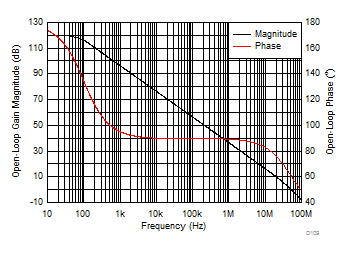Figure 44. Open-Loop Gain and Phase vs Frequency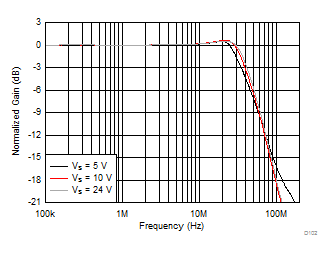See Figure 64, Gain = 2 V/V
Figure 46. Large-Signal Response vs Supply Voltage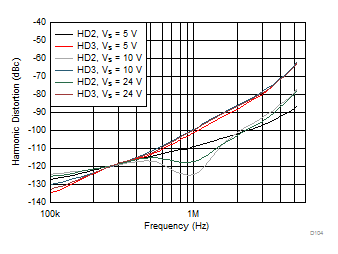See Figure 65, Gain = –1 V/V
Figure 48. Harmonic Distortion vs Frequency vs Supply Voltage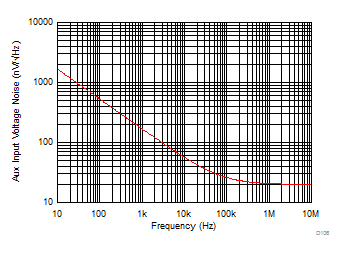Measured then fit to ideal 1/f model
Figure 50. Auxiliary Input Stage Voltage Noise Density vs Frequency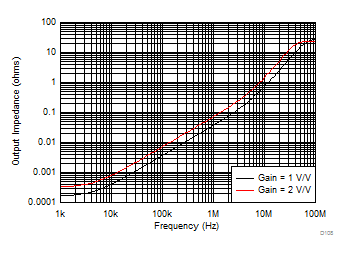See Figure 64 (simulation)
Figure 52. Closed-Loop Output Impedance vs Frequency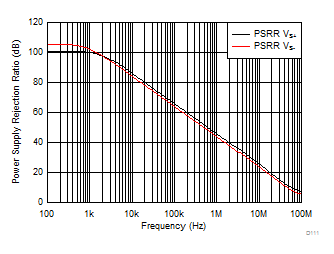Simulated Curves, VS = 5 V and 10 V
Figure 54. Power Supply Rejection Ratio vs Frequency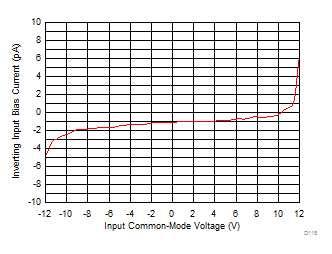VS = ±12-V
Figure 56. Input Bias Current vs Input Common-Mode Voltage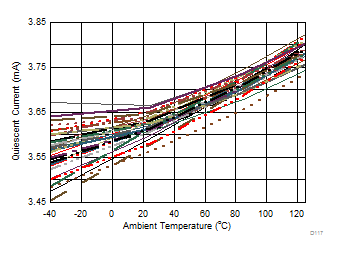70 units, DGK package
Figure 58. Quiescent Current vs Ambient Temperature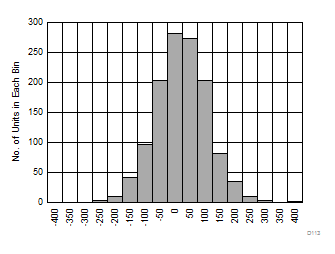Input offset voltage (µV), 1246 units
Figure 60. Input Offset Voltage DistributionSee Figure 64, Gain = 1 V/V, RF = 0 Ω
Figure 45. Large-Signal Response vs Supply Voltage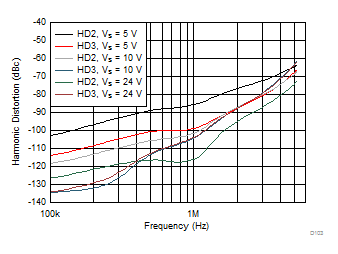See Figure 64, Gain = 2 V/V
Figure 47. Harmonic Distortion vs Frequency vs Supply Voltage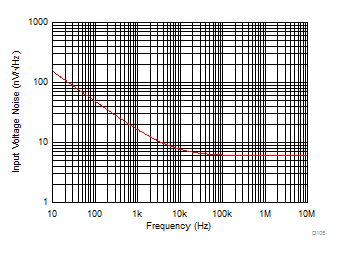Measured then fit to ideal 1/f model
Figure 49. Input Voltage Noise Density vs Frequency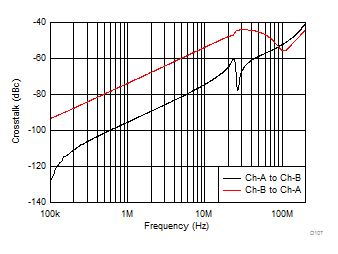See Figure 64, Gain = 1 V/V, RF = 0 Ω
Figure 51. Crosstalk vs Frequency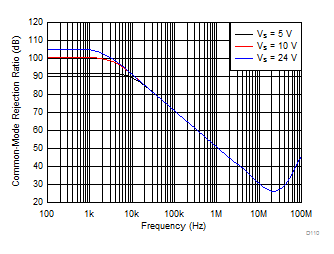Simulated curves
Figure 53. Common-Mode Rejection Ratio vs Frequency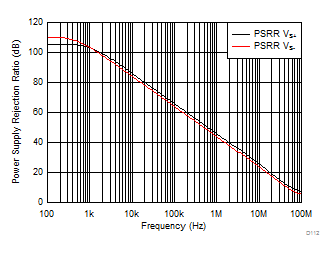Simulated curves, VS = 24 V
Figure 55. Power Supply Rejection Ratio vs Frequency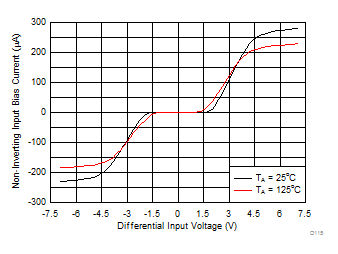Abs (VIN,Diff (max)) = VS when VS < 7 V
Figure 57. Input Bias Current vs Differential Input Voltage70 units, DGK package
Figure 59. Input Offset Voltage vs Ambient Temperature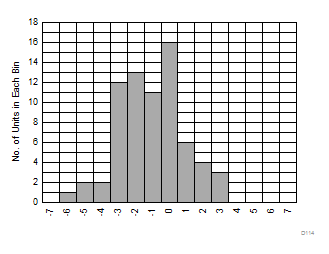Input offset voltage drift (µV/°C), –40°C to +125°C fit, 70 units
Figure 61. Input Offset Voltage Drift Distribution